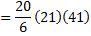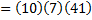# Column-I Column- II (A) If, thenis divisible by the greatest prime number which is greater than (p) 16 (B) Between 4 and 2916 is inserted odd numberG.M’s. Then thethe G.M. is divisible by greatest odd integer which is less than (q) 10 (C) In a certain progression, four consecutive terms are 40, 30, 24, 20. Then the integral part of the next term of the progression is more than (r) 34 (D)to, where H.C.F., thenis less than (s) 30 CODES :   A B C D     a) R,s p,q r,s p,q,r,s     b) p,q,r,s r,s p,q r,s     c) p,q r,s p,q,r,s r,s     d) r,s p,q,r,s r,s p,q

## Question ID - 51119 :- Column-I Column- II (A) If, thenis divisible by the greatest prime number which is greater than (p) 16 (B) Between 4 and 2916 is inserted odd numberG.M’s. Then thethe G.M. is divisible by greatest odd integer which is less than (q) 10 (C) In a certain progression, four consecutive terms are 40, 30, 24, 20. Then the integral part of the next term of the progression is more than (r) 34 (D)to, where H.C.F., thenis less than (s) 30 CODES :   A B C D     a) R,s p,q r,s p,q,r,s     b) p,q,r,s r,s p,q r,s     c) p,q r,s p,q,r,s r,s     d) r,s p,q,r,s r,s p,q

3537

 (b) 1.Hence,Hence, the greatest prime number by whichwhich is divisible is 41 2.will be the middle mean ofodd means and it will be equidistant from the first and last terms. Hence,will also be in G.P. So,Hence, the greatest odd number by whichis divisible is 27 3. Terms are 40, 30, 24, 20. Now,andHence, 1/30, 1/24, 1/20 are in A.P. with common difference. Hence, the next term is 1/20+1/120=7/120. Therefore, the next term of given series is. Hence, the integral part ofis 17 4.andColumn-I Column- II (A) Ifare in G.P., thenare in (p) A.P. (B) If, thenare in (q) H.P. (C) Ifare in A.P.;are in G.P. andare in G.P., thenare in (r) G.P. (D) Ifare in G.P.,, thenare in (s) None of these CODES : A B C D a) q r p r b) r p q s c) s r p q d) p s r q